Application Notes

# Moku:Go’s I-V Curve Tracing

Lab experiment, forward bias behavior of diode

Moku:Go combines 8 lab instruments in one high-performance device. This application note uses Moku:Go’s Oscilloscope and its integrated waveform generator to investigate the forward bias behavior of a diode.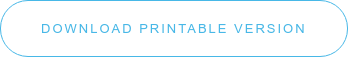## Moku:Go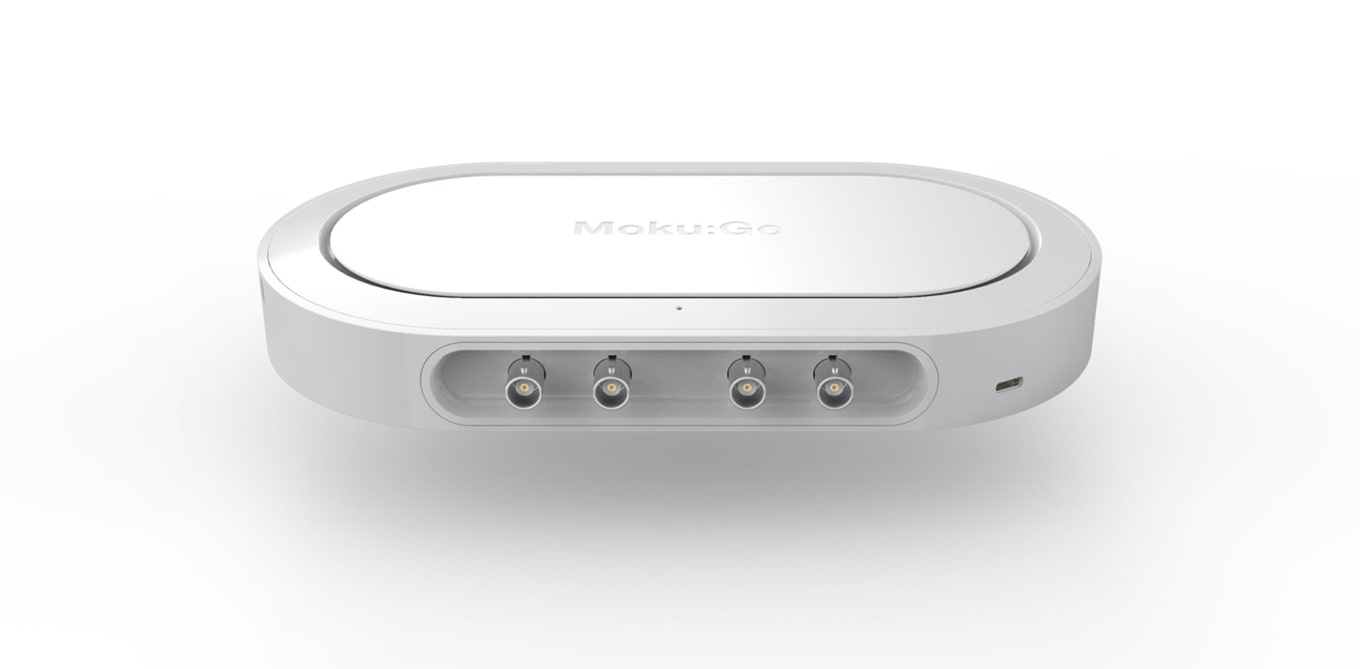Moku:Go combines 8 lab instruments in one high performance device, with 2 analog inputs, 2 analog outputs, 16 digital I/O pins and optional integrated power supplies.

## Diode P-N Junction

The diode is the simplest and most basic semiconductor device consisting of a single P-N junction. Since P-N junctions are the fundamental functional feature of many semiconductors, a strong foundational knowledge of the behavior of diodes is crucial to successful learning in more advanced lab experiments on transistors and other semiconductors.

I-V curve characterization is a fundamental measurement and common lab experiment to aid in the understanding of semiconductor junctions. I-V curves are plots of current as a function of voltage. For a resistor, the I-V curve would simply be a straight line through 0 volts, 0 amps. While dedicated I-V curve instruments exist, and some implementations use Source-Measure Units (SMUs) with appropriate software, these solutions require bulky and expensive, traditional stand-alone equipment. Here we show a diode I-V measurement using Moku:Go’s Oscilloscope and its built-in waveform generator. The captured data is shared to Excel (or alternatively MATLAB) to enable the student to manipulate the captured data and present an I-V curve for a IN4001 diode. Thus the experiment can be done with Moku:Go and no other instruments.

## Experimental setup

In order to plot an I-V curve for a IN4001 diode we have setup the circuit in Figure 1.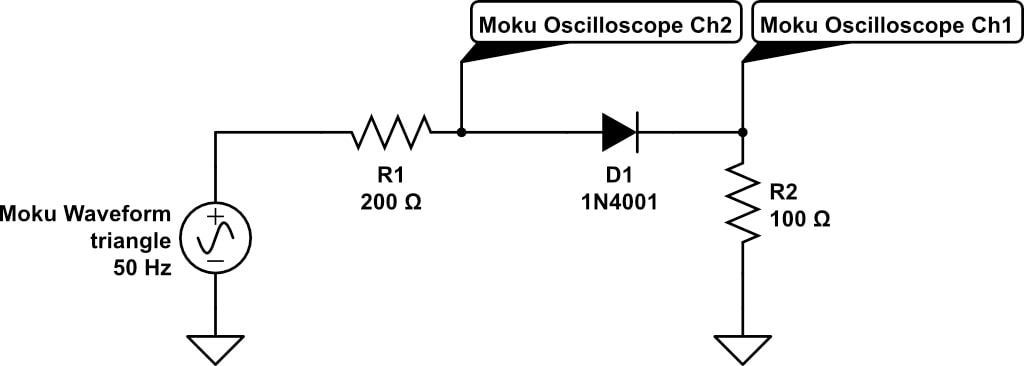Figure 1: forward bias diode circuit

R1 represents the output impedance of the waveform generator. Moku:Go’s Oscilloscope channel 2 is used to measure the voltage applied across both the diode and a current limiting & sensing resistor (R2). Then the Oscilloscope channel 1 measures the voltage across R2, a 1% tolerance 100 Ω resistor, allowing us to calculate the current through the diode.

There is a waveform generator integrated into Moku:Go’s Oscilloscope instrument. This is used to generate a triangle wave set to an amplitude of 3.2 V with 1.6 V DC offset. Thus, the diode is always forward biased and allows us to apply a swept voltage, frequency set at a low, not important 50 Hz. Figure 2 shows the waveform generator setup in the macOS app. Notice we only use waveform generator channel 1 (green); channel 2 (purple) is off. The Windows app is very similar.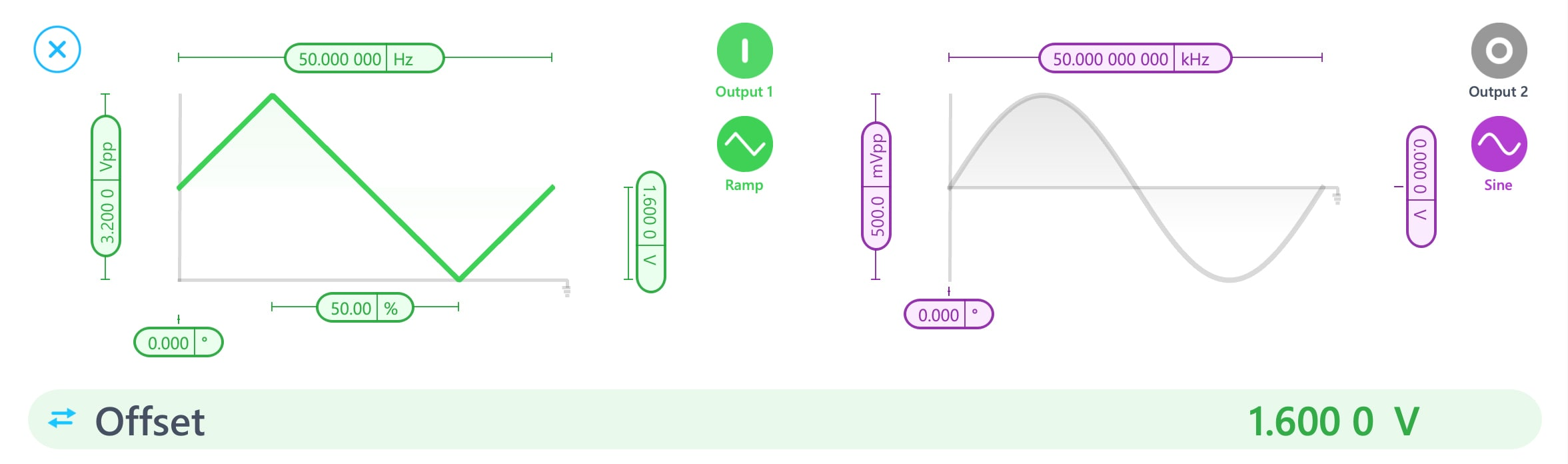Figure 2: Moku:Go’s waveform generator configured to sweep voltage

We can now use Moku:Go’s Oscilloscope to observe the voltage on channel 1 and channel 2 (refer to Figure 1 for the channel probe points). Figure 3 shows the Oscilloscope, channel A (input 1) in red, channel B (input 2) in blue and the behavior of the diode is evident.

We have also used the oscilloscope math channel to plot X-Y curve in orange and this shows the general expected I-V curve of a diode.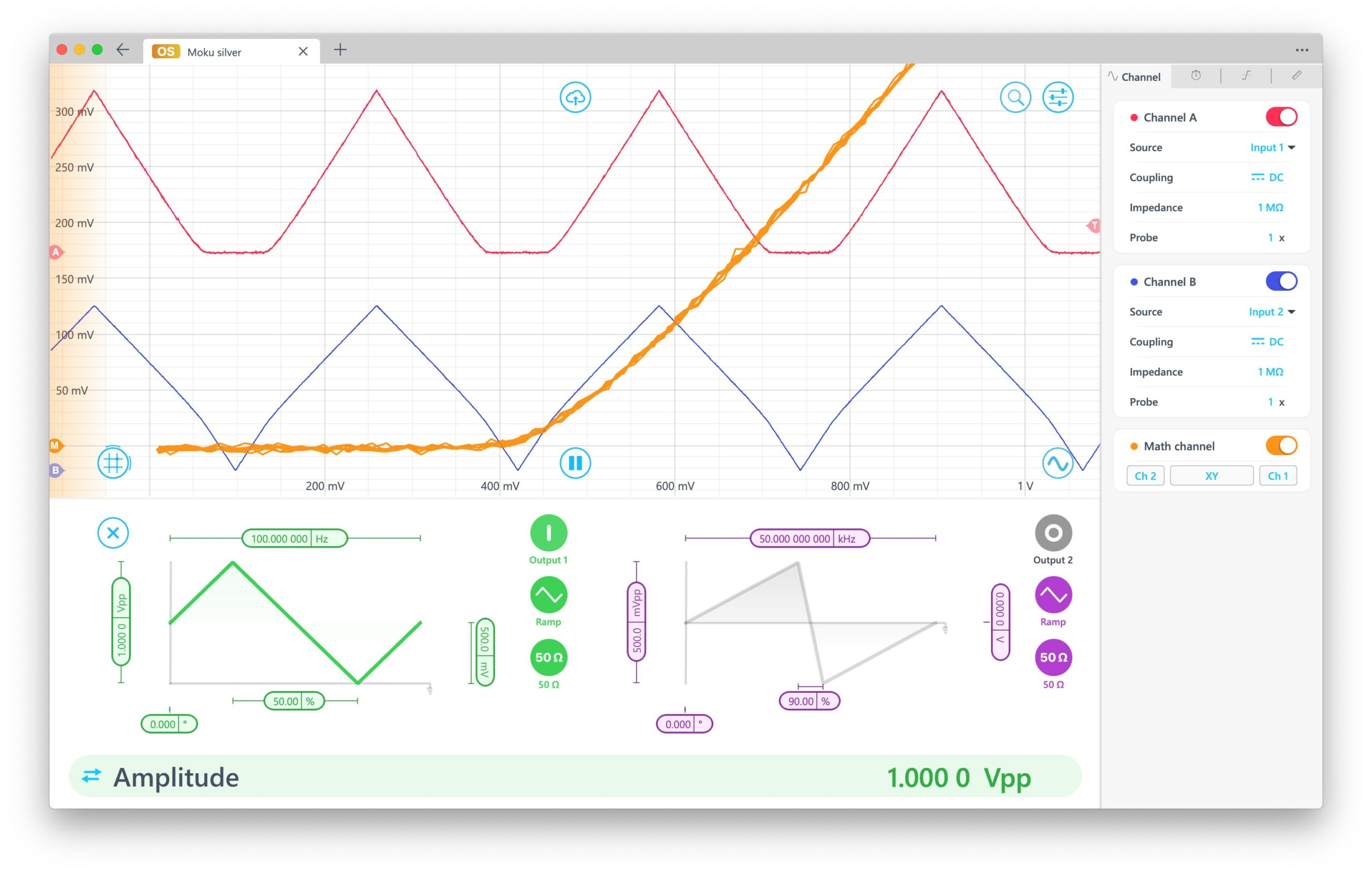Figure 3: Moku:Go’s Oscilloscope, X-Y Channel and integrated waveform generator

Referring to the circuit in Figure 1; we see that the current in the diode:

Idiode = (Vch1/100)

and that the corresponding

Vdiode = Vch2 – Vch1

Since Moku:Go is connected to the app via USB-C or the network, we can simply export the oscilloscope data to a CSV file, then calculate and plot Idiode vs Vdiode in Excel, MATLAB or similar.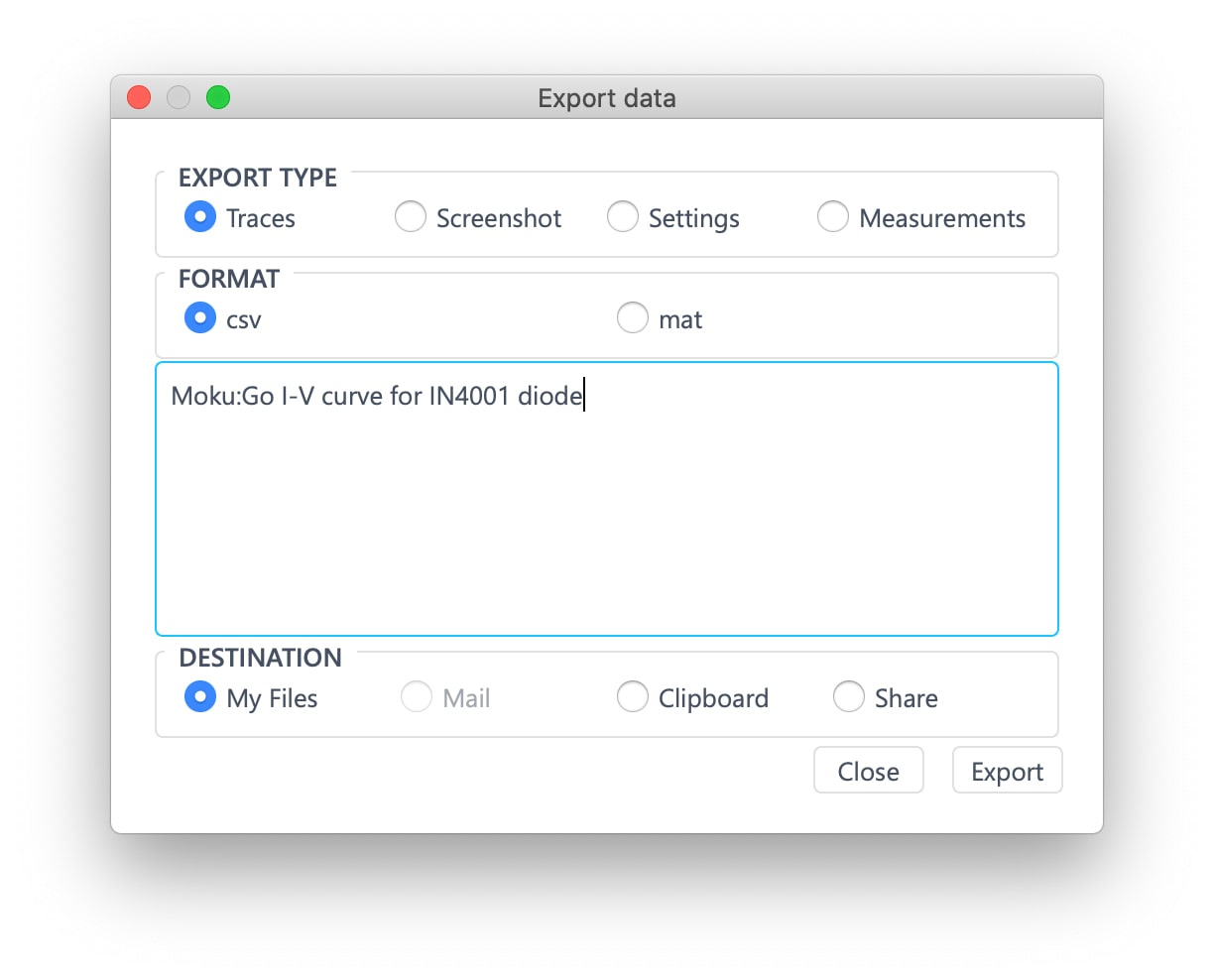Figure 4: exporting data to CSV

## Resulting I-V plot

After importing the CSV from Moku:Go into Excel, Vdiode and Idiode is calculated and plotted. The resulting I-V plot for the IN4001 diode is shown in Figure 5 exhibiting a typical forward bias turn-on voltage; after which we see large increases in current.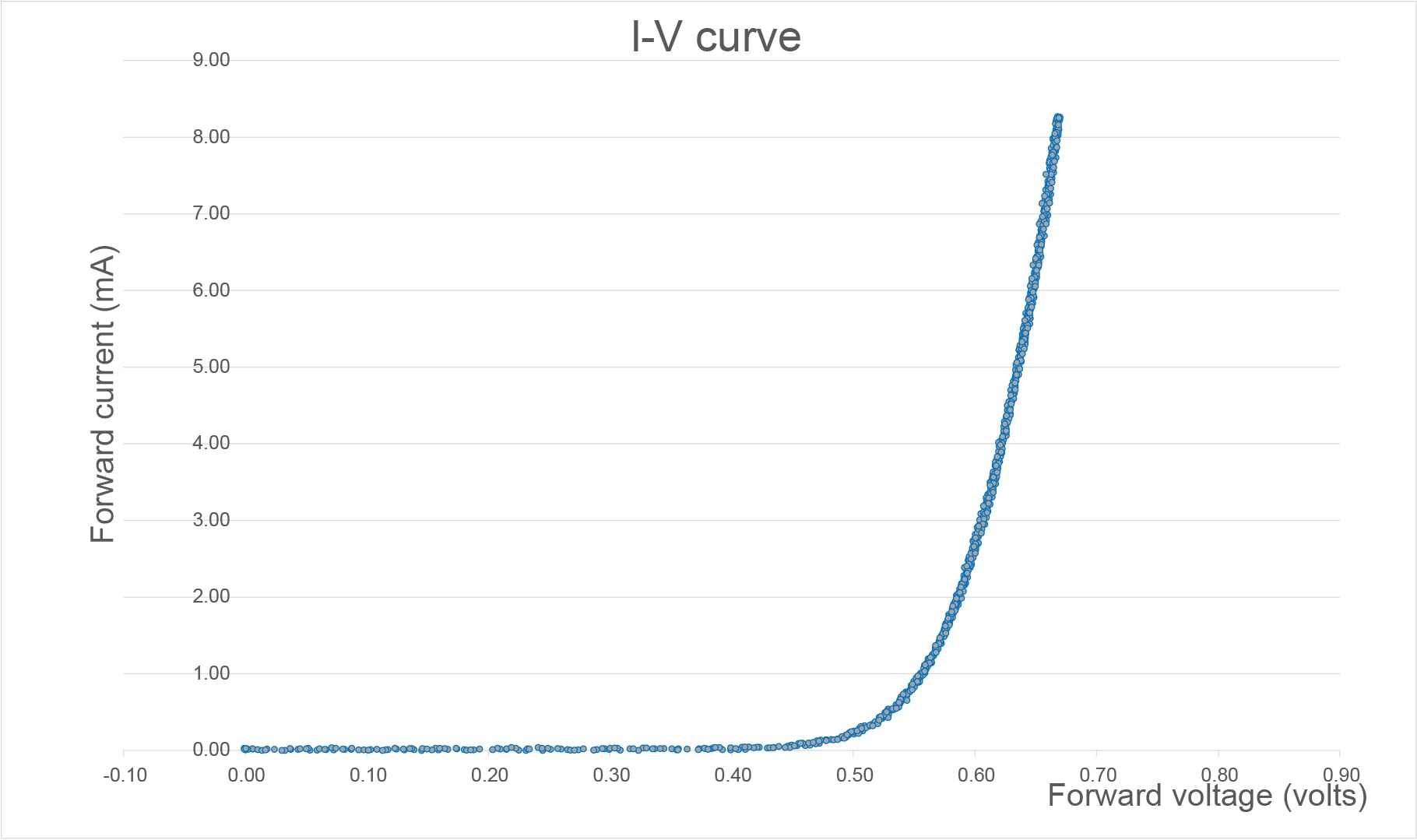Figure 5: measured I-V plot for forward biased IN4001 diode

## Summary

We have used Moku:Go and its Oscilloscope and integrated waveform generator to investigate, measure, and record the I-V behavior of a diode. This was accomplished with a simple breadboard and one Moku:Go. No other lab equipment was needed to demonstrate this common electronic engineering lab experiment.

### Benefits of Moku:Go

For the educator & lab assistants

• Efficient use of lab space and time
• Ease of consistent instrument configuration
• Focus on the electronics, not the instrument setup
• Maximize lab teaching assistant time
• Individual labs, individual learning
• Simplified evaluation and grading via screenshots

For the student

• Individual labs at their own pace enhance the understanding and retention
• Portable, choose pace, place and time for lab work be it home, on campus lab or even collaborate remotely
• Familiar Windows or macOS laptop environment, yet with professional-grade instruments

## Moku:Go demo mode

You can download the Moku:Go app for macOS and Windows. The demo mode operates without need for any hardware and provides a great overview of using Moku:Go